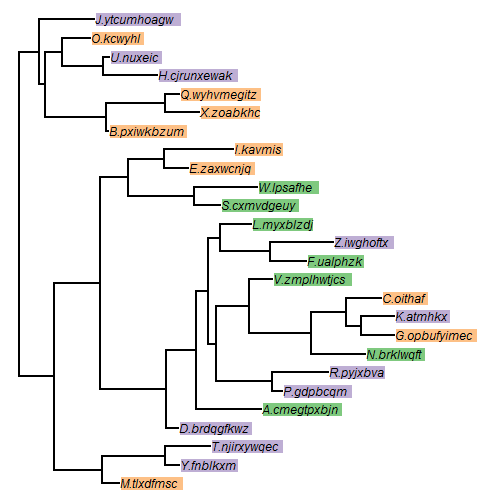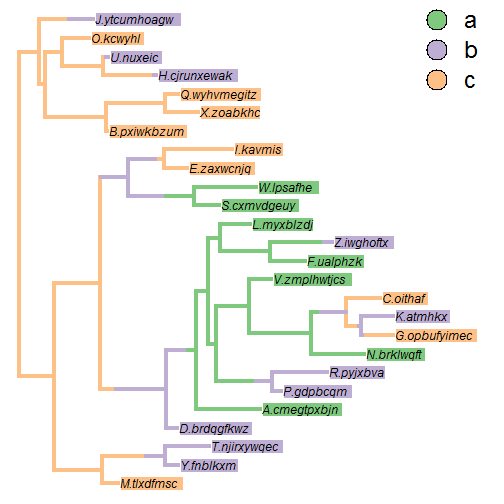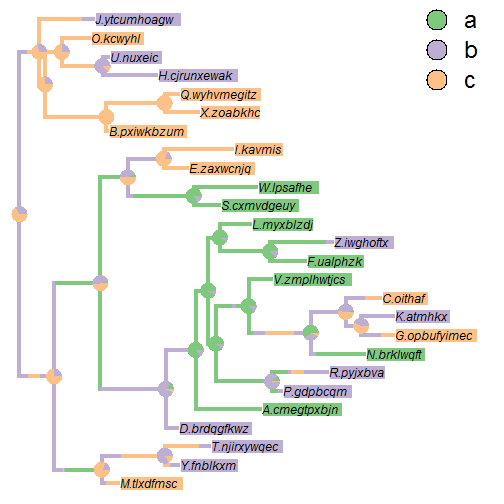## Thursday, December 14, 2017

### Drawing colored boxes around phylogenetic tip labels using R base graphics

I recently saw a post describing how to plot a tree with colored boxes around tip labels using the neat package ggtree.

Of course, it is also straightforward to do this using R base graphics. The following is just one example of how to do that:

``````library(phytools)
## custom function I'm going to use for the box labels
boxlabel<-function(x,y,text,cex=1,bg="transparent",offset=0){
w<-strwidth(text)*cex*1.1
h<-strheight(text)*cex*1.4
os<-offset*strwidth("W")*cex
rect(x+os,y-0.5*h,x+w+os,y+0.5*h,col=bg,border=0)
text(x,y,text,pos=4,offset=offset,font=3)
}
## our tree
tree
``````
``````##
## Phylogenetic tree with 26 tips and 25 internal nodes.
##
## Tip labels:
##  M.tlxdfmsc, Y.fnblkxm, T.njirxywqec, D.brdqgfkwz, A.cmegtpxbjn, P.gdpbcqm, ...
##
## Rooted; includes branch lengths.
``````
``````## our character for the colors:
x
``````
``````##   M.tlxdfmsc    Y.fnblkxm T.njirxywqec  D.brdqgfkwz A.cmegtpxbjn
##            c            b            b            b            a
##    P.gdpbcqm    R.pyjxbva   N.brklwqft G.opbufyimec     K.atmhkx
##            b            b            a            c            b
##     C.oithaf V.zmplhwtjcs    F.ualphzk   Z.iwghoftx   L.myxblzdj
##            c            a            a            b            a
##  S.cxmvdgeuy    W.lpsafhe   E.zaxwcnjq     I.kavmis  B.pxiwkbzum
##            a            a            c            c            c
##    X.zoabkhc Q.wyhvmegitz H.cjrunxewak     U.nuxeic     O.kcwyhl
##            c            c            b            b            c
## J.ytcumhoagw
##            b
## Levels: a b c
``````
``````## our colors:
cols<-setNames(RColorBrewer::brewer.pal(length(unique(x)),"Accent"),sort(unique(x)))
cols
``````
``````##         a         b         c
## "#7FC97F" "#BEAED4" "#FDC086"
``````
``````## now our plot:
par(fg="transparent")
plotTree(tree)
pp<-get("last_plot.phylo",envir=.PlotPhyloEnv)
N<-Ntip(tree)
par(fg="black")
for(i in 1:Ntip(tree)) boxlabel(pp\$xx[i],pp\$yy[i],tree\$tip.label[i],bg=cols[x[i]])
``````Of course, if the colors for the tip data come from a character purported to have evolved on the tree, perhaps we want to also show a stochastic character map of the same character evolving up the tree as follows:

``````par(fg="transparent")
plot(make.simmap(tree,x),cols,lwd=4)
``````
``````## make.simmap is sampling character histories conditioned on the transition matrix
##
## Q =
##            a          b          c
## a -0.5411151  0.5411151  0.0000000
## b  0.5411151 -1.2691823  0.7280672
## c  0.0000000  0.7280672 -0.7280672
## (estimated using likelihood);
## and (mean) root node prior probabilities
## pi =
##         a         b         c
## 0.3333333 0.3333333 0.3333333
``````
``````## Done.
``````
``````par(fg="black")
for(i in 1:Ntip(tree)) boxlabel(pp\$xx[i],pp\$yy[i],tree\$tip.label[i],bg=cols[x[i]])
``````Or maybe we want to show posterior probabilities at nodes of the tree from a set of stochastic maps:

``````trees<-make.simmap(tree,x,nsim=100)
``````
``````## make.simmap is sampling character histories conditioned on the transition matrix
##
## Q =
##            a          b          c
## a -0.5411151  0.5411151  0.0000000
## b  0.5411151 -1.2691823  0.7280672
## c  0.0000000  0.7280672 -0.7280672
## (estimated using likelihood);
## and (mean) root node prior probabilities
## pi =
##         a         b         c
## 0.3333333 0.3333333 0.3333333
``````
``````## Done.
``````
``````par(fg="transparent")
plot(trees[],cols,lwd=4)
nodelabels(pie=summary(trees)\$ace,piecol=cols,cex=0.8)
par(fg="black")
for(i in 1:Ntip(tree)) boxlabel(pp\$xx[i],pp\$yy[i],tree\$tip.label[i],bg=cols[x[i]])
``````That's pretty neat too.

The tree & data were simulated (to have realistic looking tip labels) as follows:

``````tree<-rtree(26,tip.label=LETTERS)
for(i in 1:Ntip(tree))
tree\$tip.label[i]<-paste(tree\$tip.label[i],".",paste(sample(letters,sample(6:10,1)),
collapse=""),sep="")
Q<-matrix(c(-1,1,0,1,-2,1,0,1,-1),3,3,dimnames=list(letters[1:3],letters[1:3]))
x<-as.factor(sim.history(tree,Q)\$states)
``````

1.1.